# Numerical Study of the Effect of Permeability in a Partially Porous Medium

Numerical Study of the Effect of Permeability in a Partially Porous Medium
Laboratory of Applied Energy Physics, Department of Physics, Faculty of Sciences of Matter, Batna 1University, Batna 05000, Algeria

Corresponding Author Email:
azeddine.soudani@univ-batna.dz
Page:
243-248
|
DOI:
https://doi.org/10.18280/i2m.180303
23 March 2019
|
Accepted:
5 June 2019
|
Published:
30 August 2019
| Citation

OPEN ACCESS

Abstract:

This work is devoted to the study of heat transfer in a cubic chamber partially filled with a porous material by a single fluid. The mathematical model used is that of Darcy - Brinkman - Forchheimer. The modeled equations of continuity, motion, energy and mass are numerically solved by the finite volume method. The influence of permeability and other parameters on heat transfer has been studied. The results obtained show that Nusselt numbers increase with increasing permeability for different Darcy numbers, and decrease with increasing porous thickness. The numerical results of our study are in good agreement with those found in the literature.

Keywords:

porous medium, permeability, heat transfer, natural convection, cubic cavity

1. Introduction

The effect of permeability on transfers in saturated porous media has been the subject of numerous theoretical and experimental studies. Because of their importance in many natural areas such as the movement of water in aquifers and technological like drying. The heat transfer in the porous media takes place essentially in two modes: conduction through the entire porous medium under the effect of temperature gradients; and the convection in the fluid that allows the transfer of the thermal energy it contains. The convective heat transfer is designated, according to the flow regime of the fluid, by free convection, forced convection or by mixed convection. A review of the literature shows that there is a large number of numerical and theoretical work devoted to the study of convection (forced, natural) in a porous layer. We can cite the following works:

- In 2005, Sami Ben Amara did a work on natural convection flows and heat transfer in macro-porous food environments: application to refrigerators. The approach is both experimental and numerical .

- Yacine Ould-Amer et al. are interested in natural convection in a porous multilayer square cavity. Each layer of porous medium is considered homogeneous, isotropic and saturated by a single fluid .

- For a numerical and analytical study of heat transfer by natural convection in porous layers to optimize the effect of the drag Darcy form R. Rebhi et al. have proposed the following model:

a horizontal rectangular cavity subjected to Vertical thermal gradient with transition flow governing a single-cell or multi-cell convective flux .

- Bouriche et al. also gave a numerical and analytical study on the influence of the Soret effect of natural convection developed within a porous Darcy enclosure subjected to a uniform heat flux in the presence of a magnetic field .

- Helel and Boukadida studied the transfer of heat and mass in an unsaturated porous medium subjected to laminar forced convection .

- F. Habbachi et al. are interested in the three-dimensional simulation of natural convection in a cubic cavity partially filled with porous media .

- F. Ouarhlent et al. are interested in thermal convection in a porous medium (partially porous cavity) .

Our study focuses on heat transfer caused by natural convection in a partially porous cavity . It involves studying the effect of control parameters on the flow and heat transfer mechanisms. The vertical walls of the cavity are subjected to constant temperatures. The Darcy-Brinkman-Forcheimer formulation in anisotropic saturated permeable porous medium has been retained in the present work [9-15].

2. Mathematical Formulation

The studied physical model is represented by the following geometry:

## 1.jpg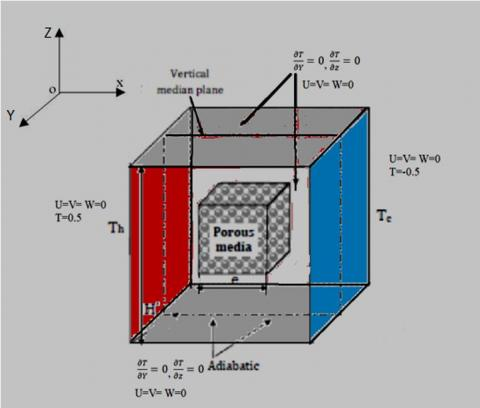Figure 1. Geometry of the studied problem

A cubic cavity of geometric parameter (H '), partially filled with a porous layer of thickness e, and saturated by a single fluid. We call η=e/H ' the dimensionless thickness of the porous layer. Vertical surfaces are subjected to Dirichlet temperature conditions, while horizontal surfaces are adiabatic (Neumann conditions).For the modeling of the problem, we adopt the following hypotheses: The fluid is Newtonian, incompressible and satisfies the Boussinesq hypothesis; the flow of fluid within the cavity is laminar and three-dimensional; it is assumed that the porous matrix is isotropic, homogeneous and in thermodynamic equilibrium with the fluid; the thermo-physical properties of the fluid are constant in the temperature range studied; and we neglect the energy transfer by radiation and by conduction. In this study, we will therefore adopt the single-domain approach of writing a single equation for the whole domain (Navier Stokes including the term Darcy), and the transition from the porous medium to the fluid medium is done by a variation of permeability ; Navier Stokes' equation including the terms Darcy-Brinkman and Forchheimer. Given the assumptions made previously, the classical conservation equations are as follows:

The continuity equation:

$\frac{\partial u}{\partial x}+\frac{\partial v}{\partial y}+\frac{\partial w}{\partial z}=0$         (1)

The amount of movement x following equation:

$\rho_{f}\left(\frac{1}{\varepsilon} \frac{\partial u}{\partial t}+\frac{1}{\varepsilon^{2}}(\vec{V} \cdot \vec{\nabla}) u\right)=-\frac{\partial p}{\partial x}+\mu_{e f f} \nabla^{2} u-\frac{\mu}{K} u-\frac{\rho_{f}}{\sqrt{k}} F|\vec{V}| u$   (2)

The amount of motion equation is following:

$\rho_{f}\left(\frac{1}{\varepsilon} \frac{\partial v}{\partial t}+\frac{1}{\varepsilon^{2}}(\vec{V} \cdot \vec{\nabla}) v\right)=-\frac{\partial p}{\partial y}+\mu_{e f f} \nabla^{2} v-\frac{\mu}{K} v-\frac{\rho_{f}}{\sqrt{k}} F|\vec{V}| v$   (3)

The amount of movement following equation z:

$\rho_{f}\left(\frac{1}{\varepsilon} \frac{\partial w}{\partial t}+\frac{1}{\varepsilon^{2}}(\vec{V} \cdot \vec{\nabla}) w\right)=-\frac{\partial p}{\partial z}+\mu_{e f f} \nabla^{2} w-\frac{\mu}{K} w-\frac{\rho f}{\sqrt{k}} F|\vec{V}| w+\rho_{f} \vec{g}$    (4)

Energy equation in porous media:

$(\rho c)_{m} \frac{\partial T}{\partial t}+(\rho c)_{f}(\vec{V} \cdot \vec{\nabla}) T=\vec{\nabla} \cdot\left(K_{e f f} \cdot \vec{\nabla} T\right)$      (5)

Or: $\rho_{f}=\rho_{0}(1-\beta \Delta T)$, β is the coefficient of thermal volume expansion of the fluid.

The adimensional formatting of the conservation equations reveals dimensionless numbers characteristic of the problem. These parameters are:

Prandtl: $P r=\frac{v}{a}$; Grashof: $G r=\frac{g \beta \Delta T L^{3}}{v^{2}}$; Rayleigh: $R a=\frac{g \beta \Delta T L^{3}}{v a}=G r . P r$.; Darcy: $D a=\frac{K}{e^{2}}$; the thickness of the porous thickness: $\eta=\frac{e}{H}$.

Finally, we express the heat transfer on active surfaces by the adimensional number of Nusselt (Nu) defined by:

$\overline{N u}=h . L / K_{f}=-\left(a\left(K_{e f f}-1\right)+1\right) \frac{\partial T}{\partial x}$         (6)

The initial and boundary conditions for this problem are:

Table 1. Terms of speed and temperature

 Condition Speed Temperature Surface H U=V= W=0 $\frac{\partial T}{\partial Y}=0, \frac{\partial T}{\partial z}=0$ Surface B U=V= W=0 $\frac{\partial T}{\partial Y}=0, \frac{\partial T}{\partial z}=0$ Surface S U=V= W=0 $\frac{\partial T}{\partial Y}=0, \frac{\partial T}{\partial z}=0$ Surface N U=V= W=0 $\frac{\partial T}{\partial Y}=0, \frac{\partial T}{\partial z}=0$ Surface W U=V= W=0 0.5 Surface E U=V= W=0 -0.5
3. Results and Discussions

In this section, we focus on the consequences of the penetration of the flow in the porous layer, as well as on heat transfer. We have placed ourselves in a porous layer in the center of the cavity, where we have varied the permeability of this layer once through the dimensionless thickness of the porous layer, which varies between 0.1 m ≤ η ≤ 0.9 m with a number Darcy's fixed, and another time through the variation of Darcy's number. The choice of an optimal mesh is not random. The independence of the mesh is a simulation test carried out with an increasingly refined mesh until the solution no longer varies with the refinement of the mesh. The geometry used is shown in Figure (2).

## 2.jpgFigure 2. Hybrid mesh of tetrahedral form

## 3.jpgFigure 3. Mesh test

The mesh test was performed on the calculation of the fields of velocities and temperatures in Figure (3). The mesh of 15625 meshes seems the optimal mesh beyond which the results are stable.

3.1 The effect of the porous layer

From Figure 4: It is found that for low permeability’s ($D a=10^{-6}$), the porous layer behaves like a solid zone where the flow is confined in this zone. The introduction of a porous layer of low permeability, causes a steep drop in the maximum velocity in the fluid zone, while in the porous zone, the velocity remains almost zero whatever η, which shows that transfers are essentially conductive in this area.

The temperature slopes in this zone indicate an increase in the thermal field. The temperature profiles show a good agreement between the present results and those of F. Habbechi Figure (5).

## 4.jpg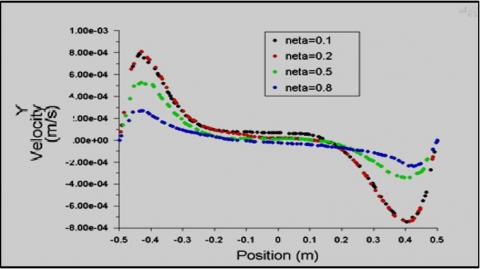Figure 4. The velocity profiles as a function of the y position and for different values of η

## 5.jpg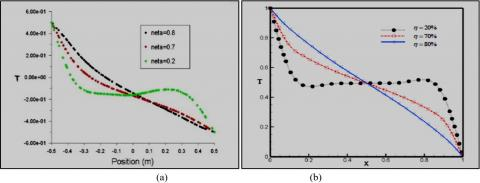Figure 5. The temperature profiles as a function of the y position and for different values of η. (a): Present study, (b): F.habbechi 

3.2 The effect of Darcy

To frame and optimize the effect of permeability on a variable Darcy number, we present numerical results, by the profiles of fluid flow and heat transfer. Along the range of the Darcy number for $\eta=20 \%$, we note that the profiles are consistent (identical and similar), because the porous layer is minimal but if it believes (η≥75 %) it fills the cavity, and in this case, we can say that:

- For low Darcy value (10-6 ≤ Da ≤ 10-4): the effect of the solid matrix is dominant with respect to the geometric complement (poral space). The porous medium behaves like a solid, which is shown in velocity trajectory at η=80 % in Figure (6-a).

-For the Darcy values (10-3 ≤ Da ≤ 1): the effect of the geometric complement (poral space) is dominant with respect to the solid matrix. The porous medium behaves like a fluid medium, which also shows the trajectory of the speed at η=80 % in Figure (6-b).

## 6a.jpg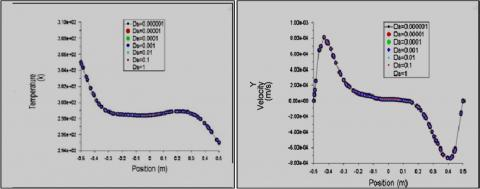(a): η =20%

## 6b.jpg(b): η=80%

Figure 6. Velocity and temperature profiles in the range $10^{-6}<D a<1$ and η = 20%  and η = 80 %

3.3 The effect of thermal transfer Nu as a function of η and Da

From Figure 6, it is concluded that the Nusselt number tends to a constant value, when the thickness of the porous layer approaches zero corresponding to pure natural convection. Similarly, as the thickness of the porous media approaches unity, the flow tends to a purely conductive situation. The porous layer has a weak effect on the natural convection flow, whose size is close to the size of the central core. Indeed, the porous medium only has a small effect on the average Nusselt number up to almost η=65 %.

Above this value of (η), the decrease is steeper and is mainly due to the suppression of the natural convection flow. Figure 6 shows three distinct zones: a first zone is purely conductive indicating a very low flow, a third zone with a convective flow for the most part indicating that the heat transfer is very important, and an intermediate zone characterized by a strong decrease in the number of Nusselt as a function of η according to the following correlation: $N u_{\text {correlation}}=-17 * \eta+14.71$.

If we notice the correlation of our study: $N u_{\text {correlation}}=-17 * \eta$+14.71is close to that of F. Habbechi gived by $N u_{\text {correlation}}=-17 * \eta$+ 15.95, with an error of 8 %. The latter is only a consequence of the computational accuracies (RESIDUAL), the nature of the mesh adopted along the study, and even the method of resolution with CFD (our study using a simple algorithm with a scheme of second order and that of Habbachi using a simple QUICK scheme algorithm).

Examination of the heat transfer effect, on fluid flow and heat transfer is illustrated by the numerical results obtained for different Darcy number values, and Figure (8) shows the evolution of heat transfer, for a wide range of Da ranging from 10-6 to 1, in the case of η = 20%, 50% and Ra = 105.

These results show that for low values of permeability (Da≥10-6), the Nusselt numbers remain practically constant; the porous medium in this Darcy range behaves as an impermeable area where flow is almost negligible. That is, conduction dominates over natural convection. For the Darcy values between: (10-6 ≤ Da ≤ 10-1), there is an increase of Nusselt, and in this zone the flow tends towards a purely convective situation through the body, and for the high values of the permeability (Da ≥10-1), the heat transfer increases further up to a certain value where the porous medium is considered as a fluid medium. If the porous layer increases (η ≥75%), it fills the cavity, in this case the Nusselt number is almost constant along the range of Da (Nu = 1.1061197 at η = 80%).

## 7.jpgFigure 7. Nusselt profile for different values of η: a. Present study, b. F.habbechi 

## 8.jpg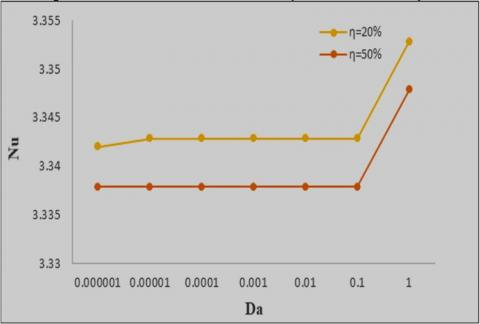Figure 8. Nusselt profile for different values of Da

4. Conclusion

We have been interested in this work in the effects of permeability on flow and transfer in porous media. The variation of the thickness of the porous layer made it possible to go from a totally fluid domain to a completely porous domain and vice versa. In our simulations, we have varied the thickness of the porous layer and the Darcy number (Da). The dependence of the flow with the various parameters of the problem has been highlighted. The existence of a porous layer of low permeability results in an extremely significant drop in heat transfer.

Finally, the influence of permeability of the porous layer on transfers was analysed. It has been found that the heat transfer coefficients increase with the permeability of the porous layer.

Nomenclature

Latin letter

$\overrightarrow{\boldsymbol{V}}$         Darcy velocity or filtration velocity (m / s);

P     Averaged magnitude of the pressure (Pa)

T     Averaged magnitude of the temperature (s);

$K_f$      Thermal conductivity of the fluid medium (W / m.K);

$K_s$      Thermal conductivity of the solid matrix (w / m.k);

$\boldsymbol{K}_{e f f}$        Effective thermal conductivity (w / m.k);

g      Gravitational acceleration (m / s2);

Empirical factor which depends on the porosity and microstructure of the porous medium(-);

(X Y Z)     dimensionless coordinate system (-);

(U, V, W) Component dimensionless speed (-);

Greek symbols

ε     Porosity of the porous medium (-);

$ρ_0$       Referential density (kg / m3);

$ρ_f$       Volumetric mass of the fluid (kg / m3);

$\boldsymbol{\mu}$      Dynamic viscosity of the fluid (kg / ms);

$\boldsymbol{\mu}_{e f f}$    Dynamic viscosity of the porous medium (kg / ms);

$\sigma$        Report of specific heats of the equivalent medium and fluid (-);

$\overset{'}{\mathop{K}}\,$ Thermal conductivity of equivalent medium (-);

$\mathbf{\eta}$      Thickness dimension without the porous layer (-);

Θ     Dimensionless temperature (-);

$\Delta \boldsymbol{T}$ Variance of temperature (S);

$\boldsymbol{\beta}$   Coefficient of thermal volume expansion of the fluid (k-1).

Indices

eff    Effective.

f       Fluid.

S      Solid.

References

 Amara, B. (2005). Ecoulements et transferts thermiques en convection naturelle dans les milieux macroporeux alimentaires applications aux réfrigérateurs ménagers. Physics. INAPG (AgroParisTech).

 Ould-Amer, Y., Slama, S. (2007). Convection naturelle dans un milieu poreux multicouche. J.Jacques, B. JITH 2007, Albi, France.

 Rebhi, R., Allichem M., Mamoum M. (2016). Étude numérique et analytique du transfert thermique par convection naturelle dans des couches poreuses. NRC Publications Archive, Archives des Publications du CNRC.

 Bourich, M., Hasnaoui, M., Amahmid, A.B.D. (2007). Effet Sorte sur la convection hydromagnétique dans une couche poreuse horizontale. JITH 2007, Albi, France.

 Helel, D., Boukadida, N. (2007). Transfert de chaleur et de masse dans un milieu poreux non saturé soumis à une convection forcée laminaire. JITH 2007, Albi, France.

 Habbachi, F., Oueslati, F.S., Bennacer, R., Ganaoui, M., Elcafsi, A. (2017). Three-dimensional simulation of natural convection in cubic cavity partially filled with porous media. Energy Procedia, 139: 617-623. https://doi.org/10.1016/j.egypro.2017.11.262

 Ouarhlent, F., Makhloufi, L. (2018). Etude numérique de la convection thermique dans un milieu poreux. Mémoire de Master, Spécialité Dynamique Des Fluides et Energétique, Université El –Hadj Lakhdar-Batna.

 Le Breton, P., Caltagironeet, J.P., Arquis, E. (1991). Natural convection in a square cavity with thin porous layers on its vertical walls. J. Heat Transfer, 113: 892-898. https://doi.org/10.1115/1.2911218

 Ouarhlent, F., Soudani, A. (2018). Etude numérique de la convection thermique dans un milieu poreux. Revue des Energies Renouvelables, 21(4): 495-504.

 Ouarhlent, F., Soudani, A. (2019). Numerical study of thermal convection in a porous medium. Instrumentation Mesure Metrologie Journal, 18(1): 69-74.

 Younsi, R., Harkati, A., Kalache, D. (2003). Numerical simulation of heat and mass transfer in composite fluid-porous layer with transverse magnetic field. Comp. Ass. Mech and Eng. Sc. J., 10(1): 57-68.

 Frederick, R.L., Moraga, S.G. (2007). Three dimensional natural convection in finned cubicle enclosures. International Journal of Heat and Fluid Flow, 28: 289-298. https://doi.org/10.1016/j.ijheatfluidflow.2006.03.005

 Tric, E., Labrosse, G., Betrouni, M. (2000). A first incursion in to the 3D structure of natural convection of air in a differentially heated cubic cavity, from accurate numerical solutions. Int. J. Heat Mass Transfer, 43: 4043-4056. https://doi.org/10.1016/S0017-9310(00)00037-5

 Fusegi, T., Hyun, J.M., Kuwahara, K., Farouk, B. (1991). A numerical study of three-dimensional natural convection in a differentially heated cubical enclosure. International Journal Heat Mass Transfer, 34(6): 1543-1557. https://doi.org/10.1016/0017-9310(91)90295-P

 Latrèche, A., Djezzar, M. (2012). Etude numérique de la convection naturelle en milieu poreux saturé de fluide dans unecavité rectangulaire à orientation variable soumise à des conditions dechauffage périodiques. The 2nd International Seminar on New and Renewable Energies Unité de Recherche Appliquée en Energies Renouvelables, Ghardaïa, Algérie.